# A Voyage to the Kernel, Day 9

0
3553

The study of secret communication systems has lured people of all ages. And the old methods of encrypting messages are quite popular even in literature. But our interest is centred around two aspects-cryptography and cryptanalysis. Cryptography, in plain words, is concerned with the design of secret communications systems, while the latter studies the ways to compromise secret communications systems!

We all know that when a bank upgrades its systems to incorporate IT, it has to make sure that the methods of electronic funds transfer are just as secure as funds transferred by an armoured vehicle.

You might have seen the arithmetic and string-processing algorithms that people employ in this realm, which are what beginners are expected to study.

Cryptanalysis, for sure, can place an incredible strain on the available computational resources. That is why people consider this to be a very tedious process. To comprehend this, let’s discuss a simple case of cryptography.

Let sender (S) send a message (called plaintext) to a particular receiver (R). ‘S’ converts his plaintext message to a secret form for transmission (which we may call the ciphertext) with the aid of a cryptographic algorithm (CA) and some defined key (K) parameters. ‘CA’ is the encryption method used here.

The whole procedure assumes some prior method of communication, as ‘R’ needs to know the parameters. The headache of the crypt analyst is that he needs to decipher the plaintext from the ciphertext without knowing the key parameters.

As we discussed, one of the simplest (and one of the oldest, too!) methods for encryption is the Caesar cipher method. Here, if a character in a particular place of the word is the Nth letter of the alphabet series, it is replaced by the (N + K)th letter in the series, where K is the parameter-an integer (Caesar used K = 3!).

```CAESAR(CA,N,K)
for_all_characters
character (N+K)? character(N)```

You can add more statements to fix bugs (say, if you’re using English, you can specify what to do if (N+K) exceeds 26).

Well, as said before, this method is very simple. Therefore, it’s no big deal for the crypt analyst to crack the encrypted data. Things will become more complex if we use a general table to define the substitution and then use the same for the process. But here, too, our villain can try some tricks. He may choose the first character arbitrarily, say E (as E is the most frequent letter in English text). He may also choose not to go for certain diagrams such as QJ (as they never occur together in English).

You can develop the method further by using multiple look-up tables. Then, you will come across many interesting cases like the one when the key is as long as the plaintext (‘one-time pad’ case) and so on. It should be noted that if the message and key are encoded in binary, a more common scheme for position-by-position encryption is to use the “exclusive-or” function to encrypt the plaintext-“exclusive-or” it (bit by bit) with the key.

## Geometric algorithms

This methodology can be adopted to solve complex problems that are inherently geometric. It can be applied to solve problems concerning physical objects ranging from large buildings (design) and automobiles, to very large-scale integrated circuits (ICs).

But, you will soon see that even the most elementary operations (even on points) are computationally challenging. The interesting aspect is that some of these problems can readily be solved just by looking at them (and some others by applying the concepts in graph theory). If we resort to computational methods, we may have to go in for non-trivial methodologies.

This branch is relatively new and many fundamental algorithms are still being developed. Hence you can consider this as a potentially challenging and promising realm.

In this introductory piece, we’ll restrict ourselves to the two-dimensional space. If you are able to properly define any point, then we can easily manage to include complex geometrical objects, say a line (as it is a pair of points connected by a straight line segment) or a polygon (defined by a set of points-array).

We can represent them by:

```type point = record x,y: integer end;
line = record pl, p2: point end;```

It is quite easy to work with pictures compared to numbers, especially when it comes to developing a new design (algorithm) pattern. It is also very helpful while debugging the code.

Let’s see a recursive program that will enable us to ‘draw’ a line by drawing the endpoints.

```procedure draw(l: line) ;
variable Δx, Δy: integer;
p: point; 10,11: line;
begin
dot(l.pl.x,l.pl.y); dot(l.p2.x,l.p2.y);
Δx:=l.p2.x-1.pl.x; Δy:=l.p2.y-1.pl.y;
if (abs(Δx)>l) or (abs(Δy)>l) then
begin
p.x:=l.pl .x+Δx div 2; p.y:=l.pl .y+Δy div 2;
ll.pl:=l.pl;   l.p2:=p; draw(l0);
l2.pl:=p; l2.p2:=l.p2; draw(11);
end ;
end```

You can see that there is a division of the space into two parts, joined by using line segments. You may stumble upon many algorithms where we will be converting geometric objects to points in a specific way. We can group them under the term ‘scan-conversion algorithms’. To get a clear picture, you may write the pseudo code to check whether two lines are intersecting. (Hint: check for a common point.)

If you can’t straight away do it, try this function to compute these lines and check whether they meet our condition:

```function same_point(l: line; pl,p2: point): integer;
variable Δx, Δy, Δxl, Δx2, Δyl, Δy2: integer;
begin
Δx:=l.p2.x-1.pl.x;    Δy:=l.p2.y-1.pl.y;
Δxl :=pl .x-l.p1.x; Δyl :=pl.y-l.p1 .y;
Δx2:=p2.x-1.p2.x; Δy2:=p2.y-1.p2.y;
same_point:=(Δx*Δyl-Δy*Δxl)*(Δx*Δy2-Δy*Δx2)
end;```

If the quantity (Δx. Δyl – Δy. Δxl) is non-zero, we can say that pl is not on the line.

## A problem for beginners

Here we are not trying to address a real problem! We will look at how to produce graphical output with the help of libraries. You might have drawn ‘pictures’ in BASIC while at school, but this is not that method. In fact, our intentions are different.

Let’s define our problem: We need to draw a sphere with the help of a few straight lines.

We can use HoloDraw (see the resource links for more information) as the library for drawing the sphere and we will do the codes in Shell. We start by ‘flattening’ the sphere to a flat rectangular map.

As it is a sphere, we will meddle with the changes in terms of ‘degrees’. We also need an input file for processing by the HoloDraw. (Before you proceed, download a copy of HoloDraw and untar it into a local directory. Also make sure that you have Perl installed.)

The input file, sphere.draw, will be quite akin to the following:

```color=0 1 0
# draw a line around the sphere’s equator
line: 0 0 1000, 360 0 1000
line: 0 45 1000, 360 45 1000
line: 0 -45 1000, 360 -45 1000

color=0 0 1

line: 0 90 1000, 0 -90 1000
line: 180 90 1000, 180 -90 1000

line: 30 90 1000, 30 -90 1000
line: 60 90 1000, 60 -90 1000
line: 90 90 1000, 90 -90 1000
line: 120 90 1000, 120 -90 1000
line: 150 90 1000, 150 -90 1000
line: 210 90 1000, 210 -90 1000
line: 240 90 1000, 240 -90 1000
line: 270 90 1000, 270 -90 1000
line: 300 90 1000, 300 -90 1000
line: 330 90 1000, 330 -90 1000```

Here the X and Y values (which you can identify from the codes directly) are in degrees around the sphere. And Z (or some axis reference) is the sphere’s radius. As you can see, we have used different colours for east-west lines and north-south lines.
Now we will create our flat grid file from this, using the following shell code:

```#!/bin/sh
/path_to_holodraw/drawwrl.pl < /location_of_input_file/sphere.draw > flatgrid.wrl```

But when we draw the sphere, we have to slice our long lines into small ones, so that our sphere will have a ‘smooth’ curve. We can do that by using the ‘drawchop’ and ‘drawball’ library files:

```#!/bin/sh
/path_to_holodraw/drawchop.pl x=15+15 y=15+15 < /location_of_input_file/sphere.draw |
/path_to_holodraw/drawball.pl |
/path_to_holodraw/drawwrl.pl > ballgrid.wrl```

We can create the VRML (Virtual Reality Modelling Language) using the ‘drawwrl’ file:

```# VRML V2.0 utf8
# draw a line around the sphere’s equator
Shape {
appearance Appearance {
material Material {
emissiveColor 0 1 0
transparency 0
}
}
geometry IndexedLineSet {
coord Coordinate {
point [
0 0 1000,
500 0 866.025403784439,
866.025403784439 0 500,
1000 0 6.12303176911189e-14,
866.025403784439 0 -500,
500 0 -866.025403784439,
1.22460635382238e-13 0 -1000,
-500 0 -866.025403784439,
-866.025403784438 0 -500,
-1000 0 -1.83690953073357e-13,
-866.025403784439 0 500,
-500 0 866.025403784438,
-2.44921270764475e-13 0 1000
]
}
coordIndex [ 0, 1, 2, 3, 4, 5, 6, 7, 8, 9, 10, 11, 12 ]
}
}

Shape {
appearance Appearance {
material Material {
emissiveColor 0 1 0
transparency 0
}
}
geometry IndexedLineSet {
coord Coordinate {
point [
0 707.106781186547 707.106781186548,
353.553390593274 707.106781186547 612.372435695795,
612.372435695795 707.106781186547 353.553390593274,
707.106781186548 707.106781186547 4.32963728535968e-14,
612.372435695795 707.106781186547 -353.553390593274,
353.553390593274 707.106781186547 -612.372435695795,
8.65927457071935e-14 707.106781186547 -707.106781186548,

-353.553390593274 707.106781186547 -612.372435695795,
-612.372435695794 707.106781186547 -353.553390593274,
-707.106781186548 707.106781186547 -1.2988911856079e-13,
-612.372435695795 707.106781186547 353.553390593274,
-353.553390593274 707.106781186547 612.372435695794,
-1.73185491414387e-13 707.106781186547 707.106781186548
]
}
coordIndex [ 0, 1, 2, 3, 4, 5, 6, 7, 8, 9, 10, 11, 12 ]
}
}
..........
...................```

This way the code goes on. (The complete code of `flatgid.wrl` is available here.
We can generalize it as :

```Shape {
appearance Appearance {
material Material {
emissiveColor x x x
transparency x
}
}
geometry IndexedLineSet {
coord Coordinate {
point [
xyz
]
}
coordIndex [x,y,z]
}
}```

…where x,y,z are local variables with respect to each reference point. And footer lines will be akin to:

```#HISTORY# /home/aasisvinayak/Documents/Desktop/holodraw.0.37/drawchop.pl x=30+30 y=30+30
#HISTORY# /home/aasisvinayak/Documents/Desktop/holodraw.0.37/drawball.pl

type [ “EXAMINE”, “FLY”, “WALK”, “ANY” ]
speed 1.0
}
#HISTORY# /home/aasisvinayak/Documents/Desktop/holodraw.0.37/drawwrl.pl```

This keeps track of the functions we employed.

[Initially, I thought of putting the generated images here, but later I felt that it was better to put the code itself because once you have the copy of the ‘drawwrl’ Perl source file, you can use it to analyse our input and the corresponding output.]
We have seen that with the help of libraries, we can generate complex codes quite easily. So you can employ such functions, libraries and black-boxes when you write the algorithms.

If you are able to achieve this, then you can straight away try geometrical algorithms.

Some evolutionary concepts: In a nutshell
Evolutionary algorithms themselves form another major branch. We will confine ourselves to some basic ideas, problem definitions and generalisations (definitions).General single-objective optimisation problem: This is defined as minimising (or maximising) f (x) subject to gi (x) ≤ 0, i = {1, . . . , m}, and hj (x) = 0, j = {1, . . . , p} x ∈ Ω. A solution minimises (or maximises) the scalar f (x) where x is a n-dimensional decision variable vector x = (x1 , . . . , xn ) from some universe Ω.

Single-objective global minimum optimisation: Given a function f : Ω ⊆ Rn → R, Ω = ø, for x ∈ Ω the value f * f (x* ) > -∞ is called a global minimum if and only if ∀x ∈ Ω : f (x* ) ≤ f (x). x* is by definition the global minimum solution, f is the objective function, and the set Ω is the feasible region of x.

Useful facts:

• The purpose of finding the global minimum solution(s) is called the global optimisation problem for a single- objective problem.
• Evolutionary multi-objective optimisation (EMO) refers to the use of evolutionary algorithms of any sort (like genetic algorithms, evolution strategies, evolutionary programming or genetic programming) to solve multi-objective optimisation problems.
• Other meta-heuristics that are being used to solve multi-objective optimisation problems include particle swarm optimisation, artificial immune systems and cultural algorithms.
• Differential evolution, ant colony, tabu search, scatter search, and memetic algorithms are other key ideas in the realm.

Key ideas:

• You must see that non-dominated points are preserved in objective space, and the associated solution points in the decision space.
• The design should be such that it should continue to allow algorithmic progress towards the Pareto Front in the objective function space.
• Maintain the diversity of points on Pareto/phenotype front (space) or of Pareto optimal solutions on decision/genotype space.
• Provide the decision maker (DM) sufficient but limited number of Pareto points for the selection (which results in decision variable values).

Please let me know if you wish to discuss these ideas more in depth.

Some ‘tree’ facts
In the last column, we discussed the use of trees. I will now list some of their properties that you can employ while designing the strategy:

• There will only be one node that connects two nodes in a tree
• If a tree has N nodes, there will be N-1 edges
• For any binary tree with N internal nodes, there are N+1 external nodes
• The height of a given full binary tree with N internal nodes is about log N / log 2
Finding (opting for) a strategy and the efficiency factor
While designing strategies it is important to consider their viability, effectiveness and efficiency. To comprehend the idea completely, consider a basic problem in quantum mechanics.

Schrödinger equation for the time-dependent wave function can be written as: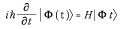We can also write an expression for the thermal expectation value of an observable X as: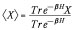You can see that the above equation is modelled by a Hamiltonian H. Classically, it is quite easy to come out with a computational method to solve such equations (say by using Monte Carlo methods). But here the problem is that the objects (say operators or matrices) in QM do not necessarily commute.

Still, we can go for models defined by: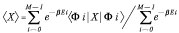A lattice of L sites filled with L/2 electrons with up spin, and L/2 electrons with down spin, is a physical model that easily fits into this. (Please Google the term ‘Hubbard model’ for more information about a better model.) But to find out what is really required to carry out these few steps, we need an order of magnitude for M. And by using approximation methods (like the Sterlings method) we can see that: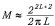This means that the quantity M increases exponentially with 2L (approximately). And if we allocate 8 bytes per floating point number, the amount of memory we need to store a single eigenvector will turn out to be: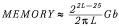So if I put L = 64, the memory required will be 1028 GB! This means that I need 1028 GB to study a quantum system of just 64 particles on 64 sites. If I submit a proposal with such high values, I am sure that no funding agency will accept this.

The only way I can do the computational task is to go for an algorithmic strategy that will reduce the amount of memory needed, at the expense of more CPU time. This is further considered in relation to ‘clouds’ and their effectiveness.

Having completed a good portion of our new segment, we can discuss the ideas you suggested. But I think it is too late to discuss notations (and advanced ideas in numerical computation) today. So wait for the forthcoming issues, in which we will address them.

### Resources